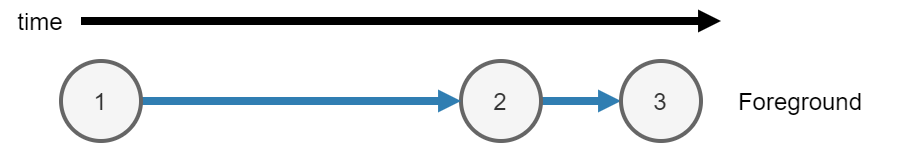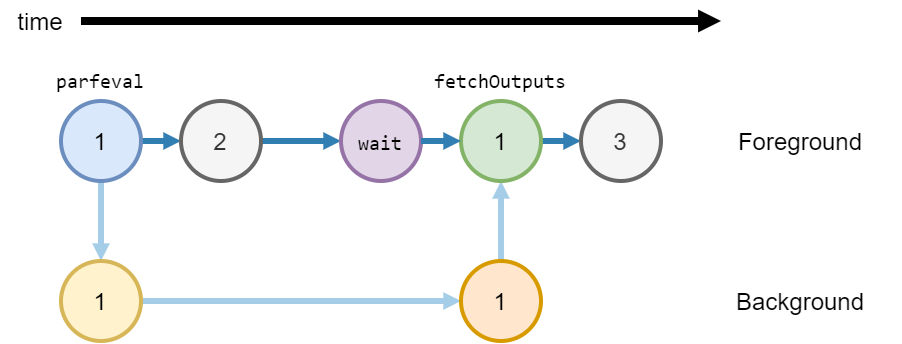## Asynchronous Functions

MATLAB® either runs code synchronously or asynchronously. You can use the following functions to run code asynchronously:

Calculate the maximum of two random matrices. MATLAB runs each line consecutively.

```tic A = rand(10000); B = ones(10000); C = max(A,B); toc```
`Elapsed time is 0.992156 seconds.`

When you run the code, MATLAB runs three calculations.

1. Calculate `A = rand(10000)` in the foreground.

2. Calculate `B = ones(10000)` in the foreground.

3. After creating `A` and `B`, calculate ```C = max(A,B)``` in the foreground.### Asynchronous Code

When you run a function asynchronously, MATLAB can run other code in the foreground at the same time.

Use `parfeval` or `parfevalOnAll` to run functions asynchronously. Use `afterEach` and `afterAll` to run functions asynchronously after a previous function completes.

Synchronous FunctionsAsynchronous Functions
MATLAB waits for the function to complete.MATLAB does not wait for the function to complete.
MATLAB runs the code immediately.MATLAB runs the code when a worker is available. For more information, see Background Workers.
Outputs from the function are available in the current workspace.To copy outputs to the current workspace, use `fetchOutputs`. When you use `fetchOutputs`, MATLAB waits for the function to complete.
You can use any function or object in a synchronous function.

You can use most functions and objects in an asynchronous function.

• Any function or object that has thread support will run in the background or on a `ThreadPool`. For more information, see Run MATLAB Functions in Thread-Based Environment.

• All functions and objects are supported on process-backed parallel pools (`ProcessPool` and `ClusterPool`). For more information, see Run Code on Parallel Pools (Parallel Computing Toolbox).

The current workspace is also available in a synchronous function.Most of the variables in the current workspace are also available in an asynchronous function.

Calculate the maximum of two random matrices: one created in the background, and one created in the foreground. Matrix `A` is created in the background, and matrix `B` is calculated in the foreground at the same time.

Note

Generally, you do not need to use `wait`. `fetchOutputs` will implicitly wait for MATLAB to finish running the function in the background before collecting results. The function `wait` is used here to explicitly show waiting for results before collecting them.

```tic fA = parfeval(backgroundPool,@rand,1,10000); B = ones(10000); wait(fA) C = max(fetchOutputs(fA),B); toc```
`Elapsed time is 0.534475 seconds.`

When you run the code, MATLAB runs three calculations.

1. Calculate `A = rand(10000)` in the background.

1. Use `parfeval` to schedule the function `rand` to run in the background, with `1` output and a single input `10000`. Return a future `fA` in the foreground.

2. Run the function `rand` in the background.

2. Calculate `B = ones(10000)` in the foreground.

3. After creating `A` and `B`, calculate ```C = max(A,B)``` in the foreground.

1. Use `wait` to explicitly wait for the future `fA` to finish running in the background.

2. Use `fetchOutputs` to get `rand(10000)` from the future `fA`.

3. Calculate the final result `C` from matrices `fetchOutputs(fA)` and `B`.### Background Workers

When you use `parfeval` or `parfevalOnAll` to run a function asynchronously, MATLAB runs the function on a pool.

When you use `backgroundPool` to run code in the background, MATLAB uses the background pool to run that code.

The background pool has a fixed number of workers. MATLAB uses these workers to run functions. Each worker can only run one function at a time. Therefore when you run multiple functions in the background, you must wait for a worker to be available to run each function.

Use the `NumWorkers` property of a `backgroundPool` to find out how many workers you have.

• If you do not have a license for Parallel Computing Toolbox, the background pool has `1` worker.

• If you have a license for Parallel Computing Toolbox, the background pool has multiple workers. The number of workers in the background pool is the number of physical cores on your machine. For example if your machine has 4 cores, the background pool has `4` workers. You can reduce this value using `maxNumCompThreads` before the first usage of `backgroundPool`.